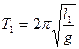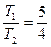# Important Subjective questions on elasticity for CBSE,JEE Main/Advanced

In this page we have Important Subjective questions on elasticity for CBSE,JEE Main/Advanced . Hope you like them and do not forget to like , social share and comment at the end of the page.
Subjective Questions
Question 1:  When a 100 Kg mass is suspended from wire of length 1 meter and and area 1 cm2, it length increases.
a) Find the stress in the wire
b) Find the increase in length of the wire
c) Find the elastic potential energy stored in the wire
d) Find the tension in the wire in loaded position
Given g=10 m/sec2 and Young Modulus of the material of the wire=2X1011 N/m2
Question 2:
A thin metallic rod of Length L and radius of cross-section r  rotates  with the angular velocity in a horizontal plane  about a vertical axis passing  through one of its end.
Density of rod is ρ and Young Modulus of elasticity is Y. Breaking stress of the rod isS
Find out the following
a) Find the tension in the rod as a function of distance from the fixed end
b) Find the tension at the fixed end, midpoint and end point of the rod
c) Find the maximum angular velocity with which it can rotate with out breaking
d) Find the total elongation in the rod

Question 3: An elastic string has a mass M suspended at its lower end,the upper being fixed to a support. When the mass is pulled down over a short distance and let go, then  it executes SHM  with Time period given byWhere l1 is the elongation of the string.Now the mass m is added to the mass M, then it is found that Time period of oscillation (T2) such that.
Find the ratio m:M
Given Y is the Young modulus of elasticity. L be the original length of string.

Solutions